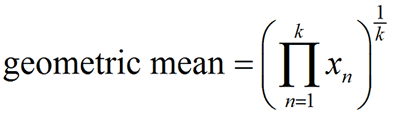# What is a Geometric Mean?

What is a geometric mean, and how is it different from a "regular" mean?

The geometric mean is not the arithmetic mean and it is not a simple average. It is the nth root of the product of n numbers. In layman's terms, that means you multiply a bunch of numbers together, and then take the nth root, where n is the number of values you just multiplied. If you multiplied two numbers, you take the square root. If you multiplied three numbers you take the cubic root.

Did that make sense? Here's a quick example:

### Example:

What is the geometric mean of 2, 8 and 4?

### Solution:

Multiply those numbers together. Then take the third root (cube root) because there are 3 numbers.

$$\sqrt{2*8*4}=4$$

As you might be expecting, the geometric mean can get very complicated. Here's a mathematical definition of the geometric mean:Remember that the capital PI symbol means to multiply a series of numbers. That definition says to multiply k numbers and then take the kth root. One thing you should know is that the geometric mean only works with positive numbers. Negative numbers could result in imaginary results depending on how many negative numbers are in a set. Typically this isn't a problem, because most uses of the geometric mean involve real data, such as the length of physical objects or the number of people responding to a survey.

Try a few more examples until you understand the geometric mean.

### Example:

What is the geometric mean of 4, 9, 9, and 2?

### Solution:

Just multiply the four numbers and take the 4th root:

$$\sqrt{4*9*9*2}=\sqrt{648}=5.045$$